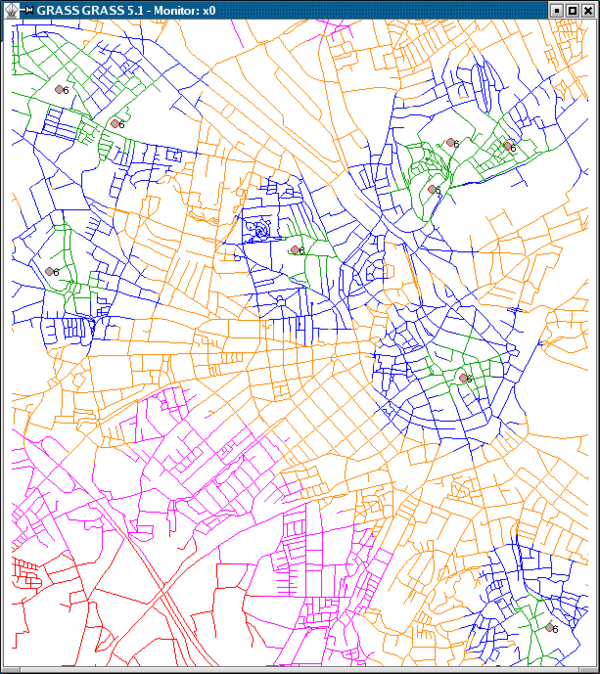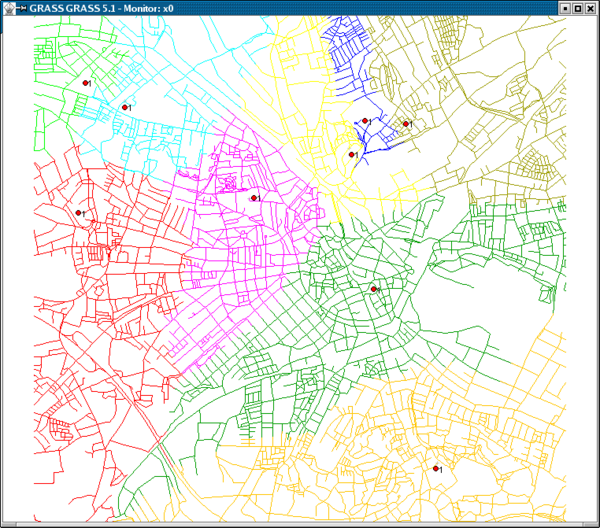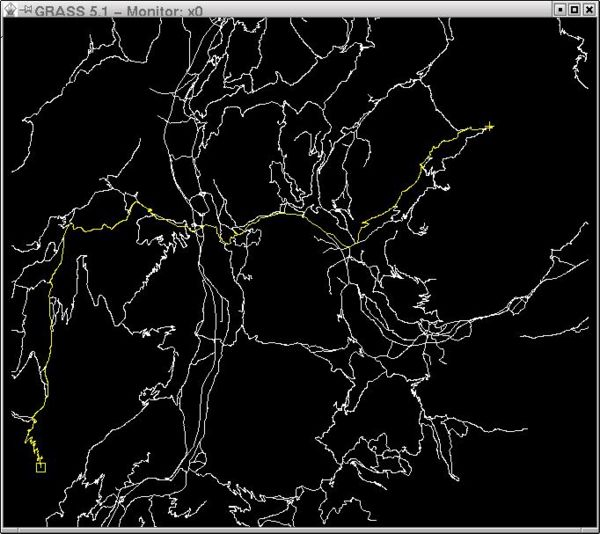# Vector network analysis

GRASS provides support for vector network analysis using the DGlib Directed Graph Library.

## Implemented algorithms

The following algorithms are implemented (GRASS 6.5+):

Vector directions are defined by the digitizing direction (a-->--b). You can navigate either omnidirectionally or differently in each directions as both directions are supported. Network modules provide parameters to assign attribute columns to the forward and backward direction. To see how a vector is directed, use the "display" parameter of d.vect (set display=dir).

• see the vectorintro "vector map processing and network analysis" help page

## Turntable extension (to be added soon)

The turntable module (v.net.turntable) is one of vector network analysis modules. It creates a turntable with the costs for every possible turn on every possible node (intersection, crossroad) in given layer. U-turns are taken in account too.

For better handling, a linegraph is created. In this linegraph, every line is represented by two nodes. These nodes have positive and negative values respectively, with their absolute values identical. Every node corresponds to opposite line direction. The positive node matches the direction of line. The negative node matches the opposite direction. For better understanding, let's have a travelling subject standing on a line (road) before an intersection wanting to cross it. This line's direction is TOWARDS the intersection. Travelling from this line through the intersection means that the subject is currently standing on the POSITIVE node representation of the line. After crossing to this line from any permitted direction, the subject gets to the NEGATIVE point representation of the line.

These two nodes (corresponding to the same line) are connected with two U-turns (for both directions). Every U-turn direction belongs to another intersection. U-turn from the POSITIVE node to the NEGATIVE one belongs to the intersection we are going to cross. The other U-turn belongs to the intersection at the opposite end of this line.

## Screenshotsv.net.iso - Split net to bands between cost isolines (direction from centre). Costs of centre node are used in calculation.v.net.alloc - Allocates subnets for nearest centres (direction from centre). Costs of centre node are used in calculation.d.path - Find shortest path for selected starting and ending node.